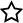How is the billing rate calculated for the time sheet report?

Updated: 11/10/2017
Article #: 97

When creating a new brand you have the option to set a billing rate, which would be used to calculate the billing rate of any ticket created under that brand.

If you were to leave the brand billing rate to \$0, the system would calculate the billing rate specified at the agent level.

Here is an example setting the billing rate per brand:

- Brand A has a Billing Rate of \$10.00 per hour.
- Brand B has a Billing Rate of \$15.00 per hour.

For the month of November,

- Brand A has 2.15 billable hours.
- Brand B has 3.00 billable hours.

The Total Amount Due for Brand A would be: \$21.50.

The Total Amount Due for Brand B would be: \$45.00.

Here is an example setting the billing rate per agent and leaving the brand rate at \$0:

- Agent 1 has a Billing Rate of \$10.00 per hour.
- Agent 2 has a Billing Rate of \$5.00 per hour.

For the month of November,

- Agent 1 has 2 billable hours
- Agent 2 has 1 billable hours

So you would take all the billable hours for each agent and multiply it by the agent's billing rate and then add them all up.

The Total Amount Due would be: \$20.00 + \$5.00 = \$25.00

Want more information on Cayzu Helpdesk, visit: www.cayzu.com

Rate this FAQ:Rating: 0.00 / Votes: 0